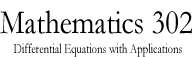Math 302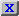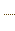HW3.pdfStatement on DisabilitiesStatement on EthicsHomeworkJava ToolsAn example of a circuitAn example of a circuitAn example of a circuitAn Application to Population DynamicsAn application to Population DynamicsSlope Field CalculatorODE 2D CalculatorODE 2D CalculatorODE 3D CalculatorODE 3D CalculatorSlope Field CalculatorSlope Field CalculatorSolution VerifierSolution Verifier 2DSolution Verifier 2DSolution VerifierA Lotke-Volterra SystemA Lotke-Volterra systemLabor Managed Oligopoly - Two firmsODE 3D Calculator2D Map CalculatorA model of sunamiA model of sunamiThe three body problemThe two body problemThe two body problemVan der Pol EquationVan der Pol EquationList of parameters of the JOde AppletBrowser test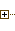Generated Documentation (Untitled)ODE 2D CalculatorUsing Marek Rychliks's Applet for Euler's MethodJOde Manual PageJOde - An Applet for Studying Ordinary Differential Equations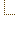ODE 2D CalculatorSyllabus
 Math Dept. Home Help room

# Solution Verifier 2D

If you have a formulas which express the solution of a system of equations
```dx/dt = f(x, y, t)
dy/dt = g(x, y, t)
```
in terms of the initial condition x=x0, y=y0 at t=t0:
```x = A(x0, y0, t0, t)
y = B(x0, y0, t0, t)
```
then you can plug it in the applet and see if blue curves (numerical solutions) coincide with red curves (obtained from your formula). You can also use this method to verify whether your formula holds for some particular initial conditions. In the example below, we compare the solution to the equation d^2x/dt^2 = t + x - x^2 to its Taylor series solution. First, this equation is re-written as a system of two equations:
```dx/dt = y
dy/dt = t + x - x^2
```
and the resulting system is fed into the applet.

View Instructions on using the Applet

Switch to unframed version

Marek Rychlik (rychlik@u.arizona.edu)